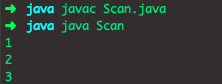# Java Arrays Example | Arrays in Java Explained

Java Arrays store a value into the variable. Now if we required more values then have to declare more variables, i.e., one variable for one value. So, here then the program will consume a lot of memory space. To resolve this problem, we have an array concept. The array is a collection of the same data types of elements and has contiguous memory allocation. You can check out ArrayList in Java article as well. Array and ArrayList are different concepts.

## Java Arrays Example

The array can contain primitives data types as well as objects of a class depending on the definition of a collection. In the case of primitives data types, the actual values are stored in contiguous memory locations. In the case of objects of a class, the real objects are stored in the heap segment.

The concept of Java array is the same just like C/C++ programming languages. We can consider Array as a data structure where we can store similar types of data.

Now accordingly we will discuss how to declare an array, initialize an array and access elements of an array. See the below figure for an array in java.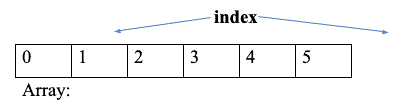The indexing of an array generally starts from 0.

But here length is of 6 as we can store here 6 elements.

First, learn how to declare an array.

We declare an array with the help of the following syntax.

```dataType []array_name; (or)
dataType array_name [];
```

Now let’s see how to allocate memory to an array if we want to allocate memory to a collection.

An array declaration has two components: the dataType and the array_name. The dataType declares the item type of an array. An element type determines the data type of each item that comprises the array.

Like an array of int types, we can also create an array of other primitive data types like char, float, double, etc. or, user-defined datatype(objects of the class). Thus, an element type for the array determines what kind of data the array will hold.

## Instantiating an Array in Java

When the array is declared, only the reference of an array is created. To create or give memory to an array, you create an array like the below. See the following syntax.

```Array_name = new dataType [Size];
```

Here we need to give the declared array name. Size is nothing but the number of elements we want to keep inside the array. If we’re going to keep 10 items the size of the array will be 10.

Note: We can declare an array and allocate memory to the declared array simultaneously with the help of the following syntax.

```dataType [] array_name = new dataType [Size];
```

Let’s talk about how to initialize elements to an array. To initialize elements, we need to follow the below syntax:

```dataType [ ] array_name = new dataType[]{
Elements which we want to add separated by coma};
```

We can also initialize elements with the help of referring to the index method.

Let’s take an example.

```int [] arr=new int ;
arr=7;
arr=10;
arr=52;
arr=5;
arr=40;
```

We can access all the array elements referring to the index number.

For example: if we want to get the vale at position 4 then

We need to print the value like arr, and the output will be 5.

Here is the full program to understand better about initializing declaring and accessing elements of an array.

```// ArrayExample.java

class ArrayExample {
public static void main(String[] er) {
int[] arr = new int;// declaring array as well as alocationg memory to the array

// initializing values with the help of index referring technique
arr = 10;
arr = 20;
arr = 30;
arr = 40;
arr = 50;
// accessing elements of the array
System.out.println("Value at index 0 or First element of the array is:" + arr);
System.out.println("Value at index 2 or Third element of the array is:" + arr);
}
}```

See the output.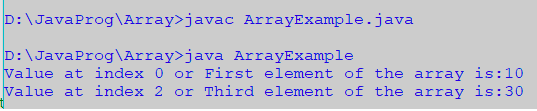## Accessing elements with the help of For loop

We can access elements using or loops. It needs length property which specifies how many times for loop should run. See the below syntax.

```for(int i=0;i<array_name.length;i++)
{
System.out.println(array_name[i]);
}
```

Using this syntax, we can access every element. Here length is the number of elements in this array.

We can even access these elements using a foreach loop. For that case, the syntax will be different.

```for (dataType i: array_name)
{
System.out.println(i);
}```

In that case, the output will be the same.

See the following code example.

```// ArrayFor.java

class ArrayFor {
public static void main(String[] er) {
int[] arr = new int;// declaring array as well as alocationg memory to the array

// initializing values with the help of index referring technique
arr = 10;
arr = 20;
arr = 30;
arr = 40;
arr = 50;
// accessing elements with help of for loop
for (int i = 0; i < arr.length; i++) {
System.out.println(arr[i]);
}

// access using the for-each loop

/*
* for (int i: arr) { System.out.println(i); }
*/

}
}```

The output is following.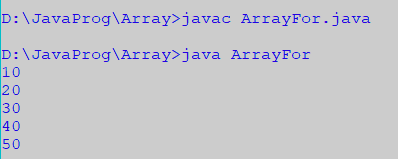Whatever examples we have discussed until now they belong to a 1D array

But there is a Multidimensional array as well. So now we are going elaborate about 2D array. How to declare it how to access it and how to initialize it. It is also known as the matrix array.

## Declaring a 2D array in Java

We can declare the 2D array using the following syntax.

```dataType[] [] array_name; or,
dataType array_name[] []; or,
dataType  []array_name[];
```

We can allocate memory to 2d array the same way we allocate memory to 1D array.

### Syntax

```int [] [] array_name =new int [Size of row] [Size of column];
```

We can initialize a 2D array just like the 1D array.

``` int [] [] arr= {{1,2,3}, {4,5,6}};
```

Or the following.

```array_name=1;
array_name=2;
array_name=3;
array_name=4;
```

Let’s take an example to understand this basic concept better.

See the following code example.

```// TwoDimensionalArray.java

import java.util.Scanner;

class TwoDimensionalArray {
public static void main(String[] er) {
Scanner sc = new Scanner(System.in);
int[][] arr = new int;// declaring array as well as alocationg memory to 2D array

// initializing values with the help of index referring technique
/*
* arr=1; arr=2; arr=3; arr=4; arr=5; arr=6;
* arr=7; arr=8; arr=9;
*/

// we can also initialize this way

/* int [] [] arr=new int[][]{{1,2,3},{4,5,6},{7,8,9}}; */

// we can also take input from the user
for (int i = 0; i < 3; i++) {
for (int j = 0; j < 3; j++) {
System.out.print("a[" + i + "][" + j + "]=");
arr[i][j] = sc.nextInt();
}
}

// accessing elements of 2D array with help of for loop matrix form
for (int i = 0; i < 3; i++) {
for (int j = 0; j < 3; j++) {
System.out.print(arr[i][j] + " ");
}
System.out.println();
}
}
}```

See the output.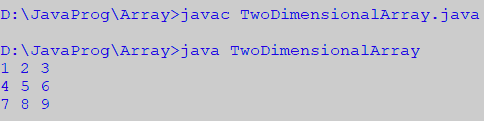## Jagged Arrays or Multidimensional Arrays in Java

It’s a special kind of Multidimensional array. Because it has the variable number of columns in each row.

To declare a Jagged array, we have to declare several rows first. The column number is different for each row so, we need to leave the column field blank while declaring any Jagged array. We can customize the number of columns as our requirement.

### Syntax

```dataType arr [] [] = new dataType [size of row] [];
```

Next point we will declare the size of a column like the following.

arr=new dataType[size of columns];

arr=new dataType[size of columns];

arr=new dataType[size of columns];

|
|
|

arr[size of row – 1]=new dataType[size of columns];

See the below picture.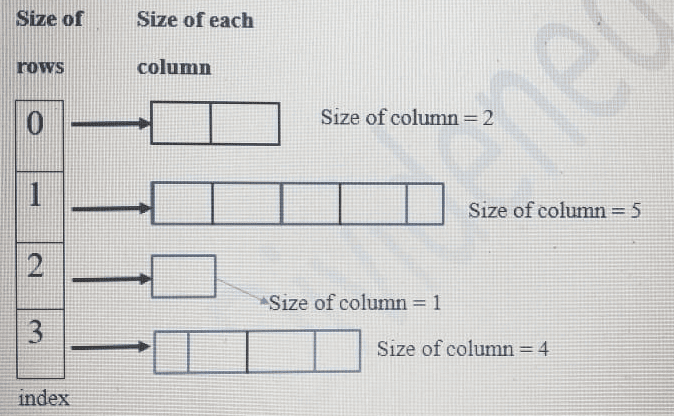See the following code of Jagged Array in Java.

```// JaggedArray.java

import java.util.Scanner;

//declaring class for jagged array
class JaggedArray {
public static void main(String[] er) {
Scanner sc = new Scanner(System.in);
// decalring jagged arrya with row size 5
int[][] arr = new int[];

// decalring column size for rows by user
System.out.println("Enter the size of the column corresponding of the row");
for (int i = 0; i < 5; i++) {
System.out.println("arr[" + i + "]=");
int s = sc.nextInt();
arr[i] = new int[s];
}

// Initializing jagged array
System.out.println("Enter elements in jagged array:");
for (int i = 0; i < arr.length; i++) {
for (int j = 0; j < arr[i].length; j++) {
System.out.println("arr[" + i + "][" + j + "]=");
arr[i][j] = sc.nextInt();
}
}

// printing jagged array
System.out.println("Elements in jagged array are:");
for (int i = 0; i < arr.length; i++) {
for (int j = 0; j < arr[i].length; j++) {
System.out.print(arr[i][j] + " ");
}
System.out.println();
}
}
}```

See the output.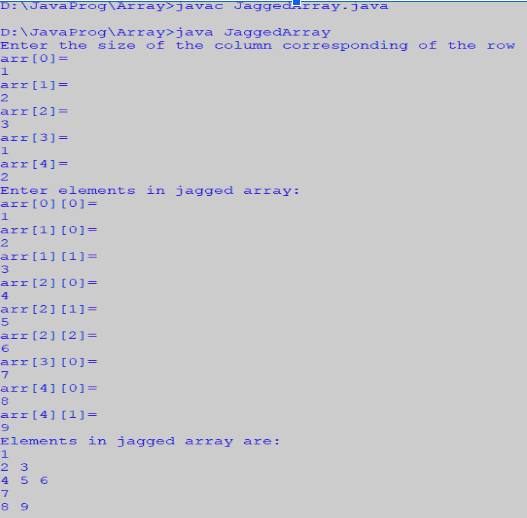Now we will see how to pass an array to Method in Java. Like variables, we can also pass arrays to methods. For example, below program pass array to method sum for calculating the sum of array’s values.

```// PassArray.java

import java.util.Scanner;

//creating a class
class PassArray {
// creating a method which passes the array as a parameter
static void max(int arr[]) {
int max = arr;
for (int i : arr) {
if (max < i)
max = i;
}
System.out.println("maximum of array is: " + max);
}

public static void main(String[] er) {
Scanner sc = new Scanner(System.in);
int n;
System.out.print("Enter size of array: ");
n = sc.nextInt();
// creating array
int arr[] = new int[n];
// taking inputs from user
System.out.println("Enter elements of array: ");
for (int i = 0; i < arr.length; i++) {
System.out.print("arr[" + i + "]=");
arr[i] = sc.nextInt();
}
max(arr);// calling method
}
}
```

See the output.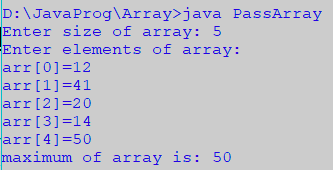### What happens if we try to access item outside the array size

Compiler throws ArrayIndexOutOfBoundsException to indicate that array has been obtained with an illegal index. The index is either negative or greater than or equal to the size of an array.

See the output.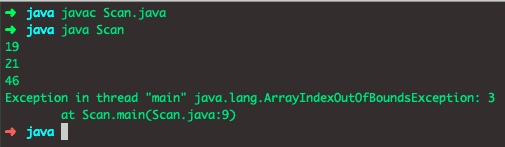## Returning Arrays from methods

As usual, any method can return an array. For example, the below program returns an array from method m1.

```// Scan.java

class Scan {
// Driver method
public static void main(String args[]) {
int arr[] = mul();

for (int i = 0; i < arr.length; i++)
System.out.print(arr[i] + "\n");

}

public static int[] mul() {
// returning array
return new int[] { 1, 2, 3 };
}
}```

See the output.